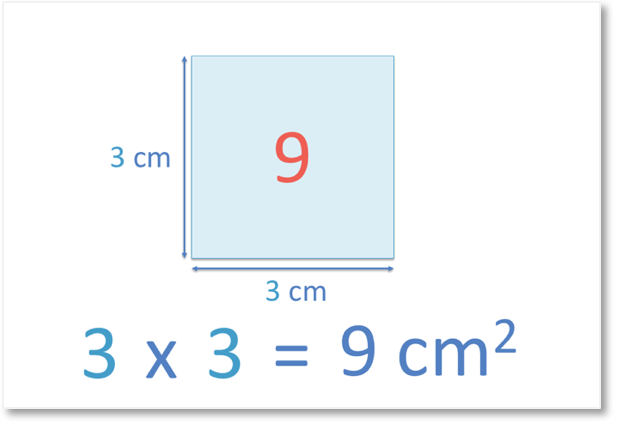# Finding Prime Numbers to 100

Finding Prime Numbers to 100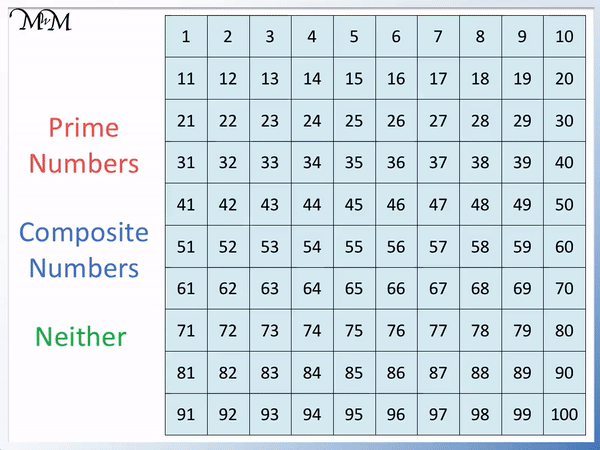• The grid above shows the prime numbers to 100.
• Prime numbers cannot be made by multiplying other smaller whole numbers.
• Prime numbers can only be written as 1 × themselves.
• We say that a prime number has exactly 2 factors, which are the number 1 and the number itself.
• Apart from 2 and 5, the other prime numbers only end in a 1, 3, 7 or 9.
• A number is not prime if it is in the times table of another number.

The prime numbers to 100 are: 2, 3, 5, 7, 11, 13, 17, 19, 23, 29, 31, 37, 41, 43, 47, 53, 59, 61, 67, 71, 73, 79, 83, 89 and 97.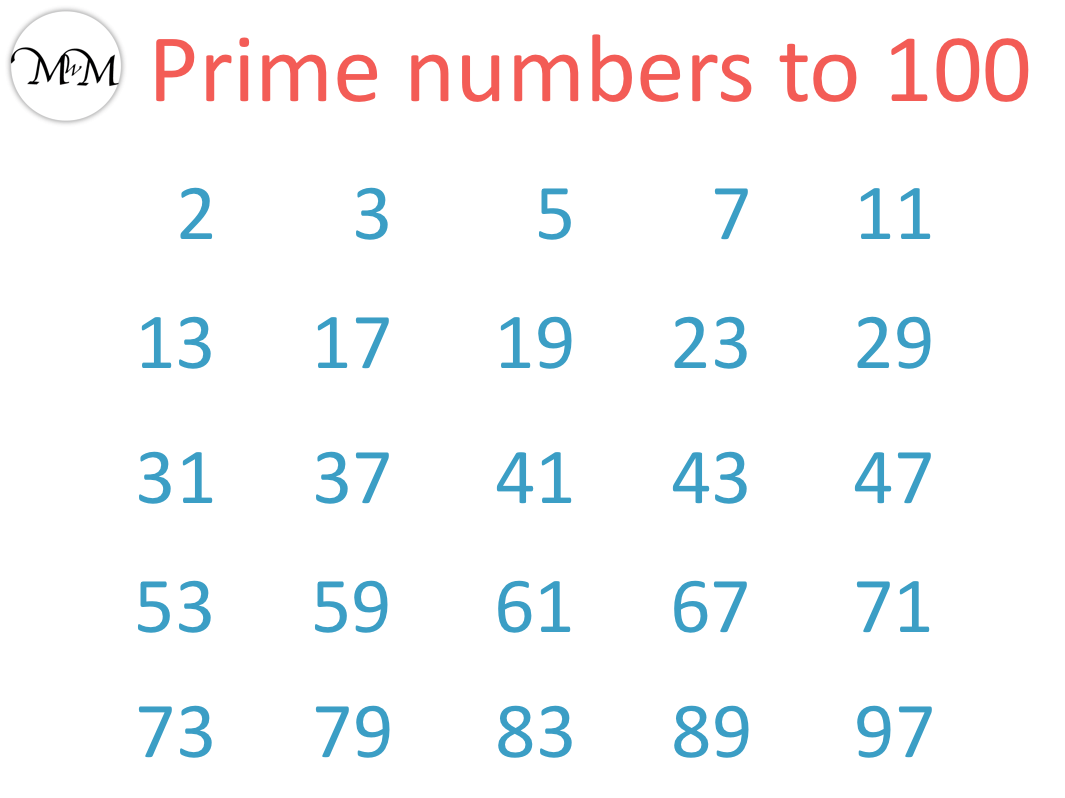Prime numbers cannot be made by multiplying 2 other smaller whole numbers.

Apart from 2 and 5, prime numbers only end in a 1, 3, 7 or 9.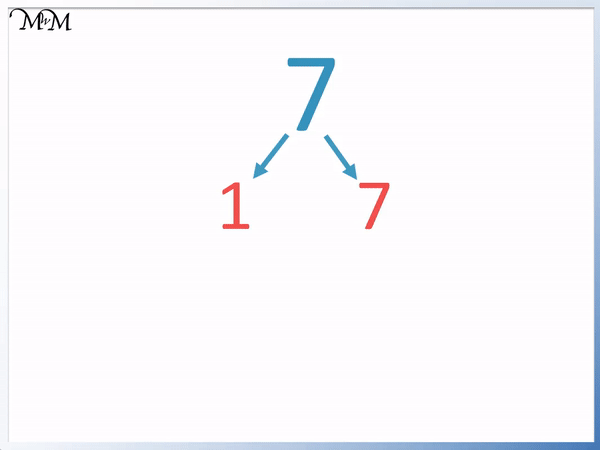• 7 is an example of a prime number.
• 7 cannot be made by multiplying two smaller whole numbers together.
• 7 can only be written as 1 × 7.
• We cannot divide any other whole number into 7 exactly.
• We can try dividing by 2, 3, 4, 5 or 6 and see that these numbers do not go into 7 exactly.# Prime Numbers to 100

## Prime and Composite Numbers

A composite number can be made by multiplying two smaller whole numbers together.

For example 6 can be made from 2 × 3.We can say that composite numbers can be broken down into other whole numbers.

Both 2 and 3 are different numbers to 6 and they are both smaller.

Because 6 can be broken down, we say that it is a composite number. It is composed of 2 times 3. 6 is in the two times table and 6 is in the three times table.

Not all numbers can be made by multiplying two smaller whole numbers.

Prime numbers are numbers that cannot be made by multiplying two smaller whole numbers.

Prime numbers are not in the times tables of any other numbers.

7 is an example of a prime number.

The word prime also means ‘first’. A prime number is the first number in a times table as long as it does not appear in another times table.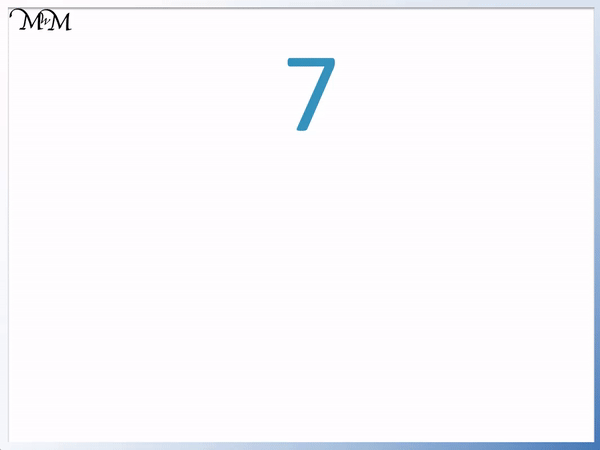7 is not in any other times table apart from the 7 times table.

7 can only be written as 1 × 7. It cannot be broken down into any smaller whole numbers.

Factors are the numbers that multiply together to make a larger number.

7 only has two factors, 1 and 7.We say that prime numbers have exactly two factors, the number 1 and the number itself.

Apart from the number 1, every number is a prime number or a composite number.

A number that is not a composite number is prime number and a number that is not a prime number is a composite number.

The number 1 is neither prime nor composite.

Prime numbers are not easy to find or identify.

The prime numbers to 100 are: 2, 3, 5, 7, 11, 13, 17, 19, 23, 29, 31, 37, 41, 43, 47, 53, 59, 61, 67, 71, 73, 79, 83, 89 and 97.## How to Find Prime Numbers

For a number to be prime it cannot be a multiple of any other smaller whole number.

To decide if a number is prime, divide it by all smaller prime numbers.

If any of the other smaller prime numbers divide into our number exactly, then it is not prime.

If all of the smaller prime numbers do not divide exactly into our number, then it is prime.

Use the following rules to decide if a number is prime:

• Apart from 2 and 5, all prime numbers will end in 1, 3, 7 or 9.
• Apart from 2 and 3, all prime numbers are one more or one less than a number in the 6 times table.
• A prime number won’t be in the times table of any other prime number which is smaller than the square root of the number we are checking.

In this lesson we will look further at these rules using some examples.

We will first look at the number two. Two is the only even prime number.2 can only be written as 1 × 2.

A prime number is the first number in a times table. 2 is the first number in the two times table and therefore it is prime.

This means that all other numbers in the two times table are not prime.

It is easy to check if a number is in the two times table. All numbers in the two times table end in a 2, 4, 6, 8 or 0.

So if a number ends in a 2, 4, 6, 8 or 0, it is in the two times table and therefore is not a prime number.

For example 4 is 2 × 2 and so, it is not prime.6 is 2 × 3 and so, it is not prime.8 is 2 × 4 and so, it is not prime.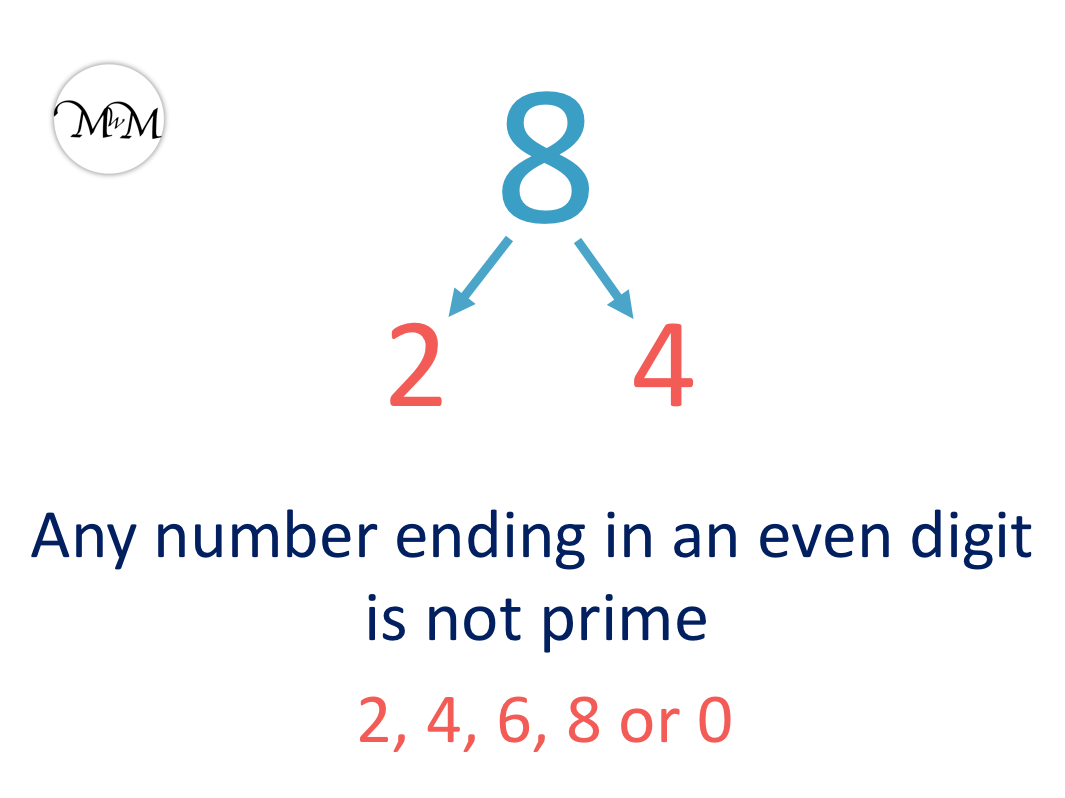10 is 2 × 5 and so, it is not prime.All numbers that end in a 2, 4, 6, 8 or 0 are in the two times table and are not prime. This rule applies to all numbers, no matter how large.

20 ends in a zero and therefore it is in the two times table and is not prime.82 ends in a 2 and so, is in the two times table and is not prime.Here are the prime numbers to 100.

We can see that apart from 2 and 5, all other prime numbers end in a 1, 3, 7 or 9.From the digits 1, 2, 3, 4, 5, 6, 7, 8, 9 and 0, we can already remove the digits of 2, 4, 6, 8 and 0 because these are numbers in the two times table.

We are left with 1, 3, 5, 7, 9 and 0.

Every number ending in 5 or 0 is also in the 5 times table and will never be prime.

This leaves us with the digits of 1, 3, 7 and 9.Not all numbers ending in 1, 3, 7 and 9 are prime. For example 99 is 9 × 11 and so is not prime.

However, this rule allows us to easily check if a number is not prime.

For example 35 ends in a 5.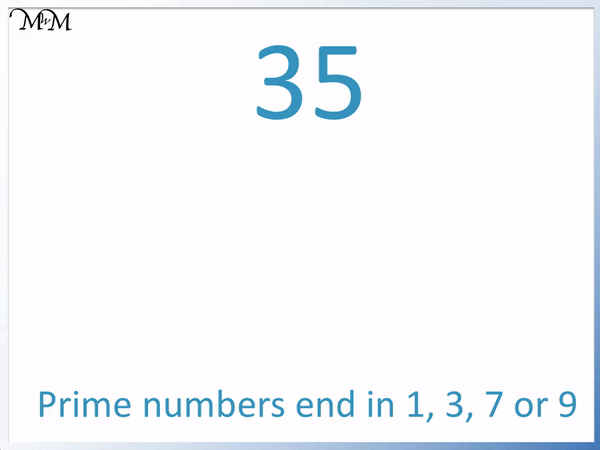We know that apart from 2 and 5, larger prime numbers only end in a 1, 3, 7 or 9. 35 ends in a 5 and so, it cannot be prime.

We will now put all of our prime numbers to 100 on a number grid.There is no pattern to the prime numbers so they can be hard to find.We can see that apart from 2 and 3, all of the prime numbers are immediately next to a number in the 6 times table.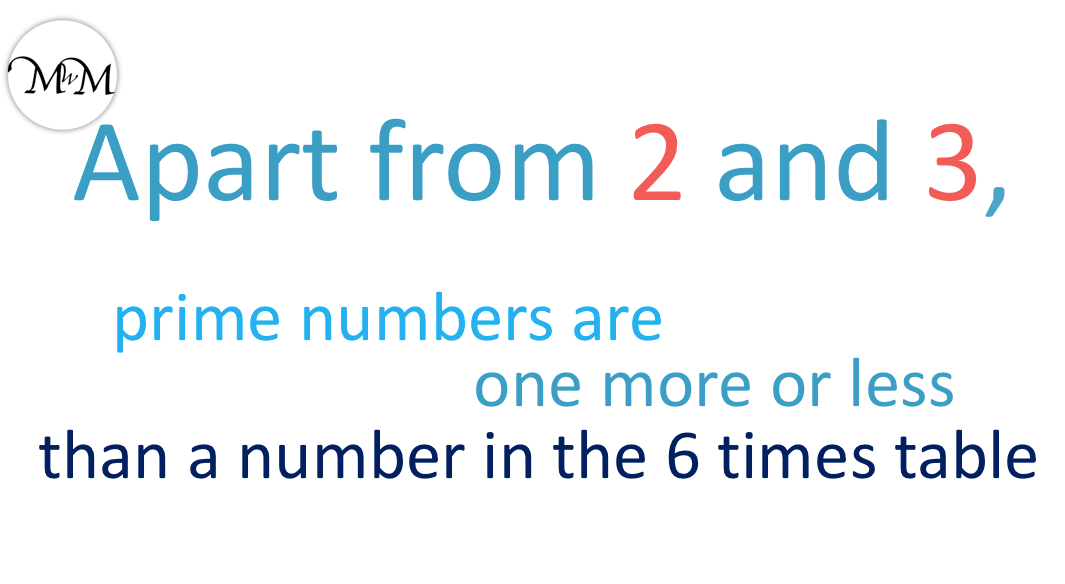All prime numbers larger than 3 are one more or one less than a number in the 6 times table.

Any number in the six times table plus 2 or plus 4 will still be even and therefore will be in the two times table.

Any number in the six times table plus 3 is still in the three times table.

This leaves the numbers that are one more or one less than a number in the six times table.

For example, 43 is a prime number. It is one more than 42, which is in the six times table.

59 is also a prime number. It is one less than 60, which is in the six times table.

This means that if a number is next to a number in the six times table and also ends in a 1, 3, 7 or 9, it is very likely to be a prime number.

However, not all numbers that end in 1, 3, 7 and 9 are prime numbers.

Here are some exceptions to be aware of.

49 is 7 × 7 and so it is not prime, even though it ends in a 9.77 is 7 × 11 and so it is not prime, even though it ends in a 7.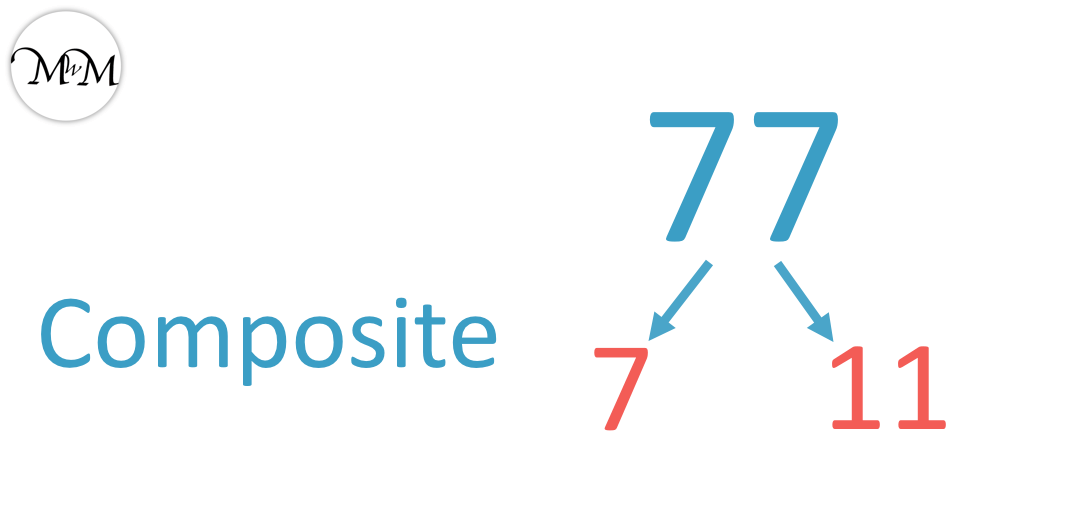91 is 7 × 13 and so it is not prime, even though it ends in a 1.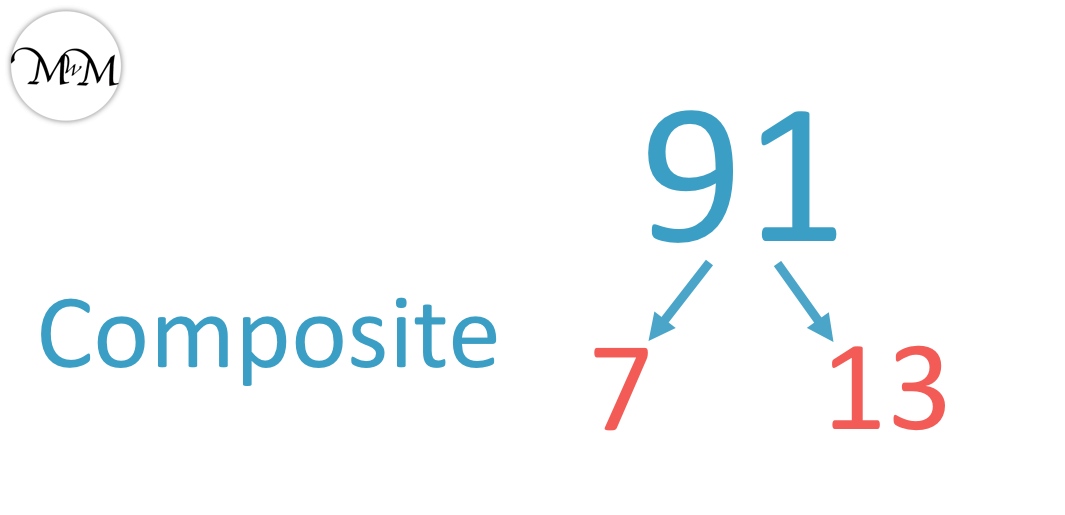So if a number ends in 1, 3, 7 or 9 and it is one more or less than a number in the 6 times table, then it might be prime.

We still need to check each number to see if it really is prime.

A prime number cannot be in the times table of any other smaller number and therefore, it cannot be divided exactly by any other smaller number.

We can divide smaller numbers into our prime number to check if they divide in exactly or not.

Fortunately we do not need to try dividing by every smaller number.

We only need to try dividing by prime numbers that are smaller than the square root of out number.

To check if a number is prime, divide by prime numbers less than the square root of the number.

The square root is the number that multiplies by itself to give the original number.

Here we have the number 31. It ends in a 1 and it is one more than 30, which is in the six times table. It might be prime and so we will test it.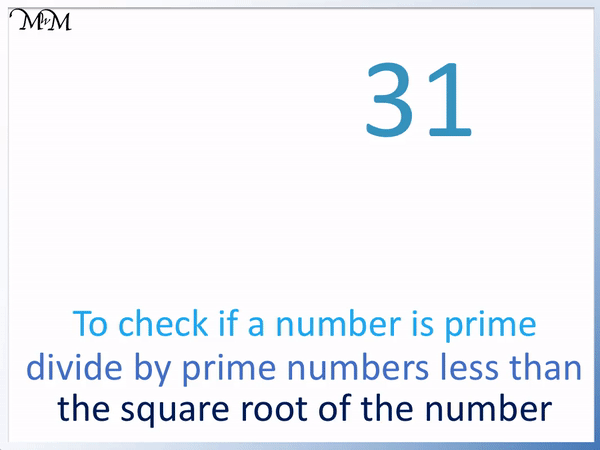We do not know the square root of 31 but we know that 6 × 6 = 36. The square root of 36 is 6 and so we will try dividing by the prime numbers less than 6.

These are 2, 3 and 5. We divide 31 by 2, 3 and 5. If 2, 3 or 5 divide exactly into 31, then 31 is not prime. If they do not divide exactly, then 31 is prime

31 is odd and so 31 cannot be divided exactly by 2.

31 also cannot be divided by 3 or 5 exactly.

Therefore 31 is prime.

Here is an example of deciding if 27 is prime.

We try to divide by prime numbers smaller than the square root of the number.

We do not know the square root of 27 but we know that 5 × 5 = 25. The square root of 25 is 5, so we will try dividing by 2, 3 and 5.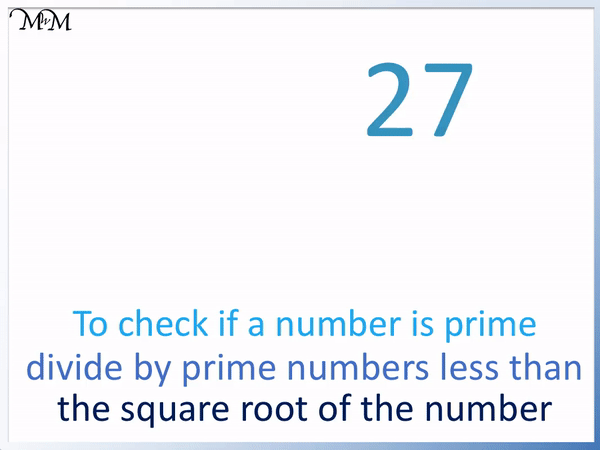27 cannot be divided by 2 because it is odd.

27 can be divided by 3. 27 ÷ 3 = 9.

We can also tell that 27 is divisible by three by adding the digits. 2 + 7 = 9, which is in the three times table and so 27 is in the three times table.

27 is not prime.

You may have noticed that 27 is also not one more or less than a number in the 6 times table and therefore is not prime.

Here is an example of 19, which ends in a 9 and is one more than 18, which is in the 6 times table.

We do not know the square root of 19 but 5 × 5 = 25, so we can try dividing by prime numbers less than 5.19 cannot be divided by 2 because 19 is odd.

19 is not in the 3 times table because 1 + 9 = 10, which is not in the three times table.

19 is not in the 5 times table because it does not end in a 5 or 0.

Because 19 is not divisible by 2, 3 or 5, it is a prime number. We have tried dividing by all of the other prime numbers less than the square root of 19.

Here is 61, which ends in a 1 and is one more than 60.

We know that 8 × 8 = 64, so we can divide 61 by all of the prime numbers less than 8.

We will divide 61 by 2, 3, 5 and 7.61 is odd and so, does not divide by 2 exactly.

6 + 1 = 7, which is not in the three times table and so, 61 is not in the three times table.

61 ends in a 1. To be in the 5 times table, a number must end in 5 or 0 and so 61 is not divisible by 5.

61 is not divisible by 3. You might know that 63 is 7 × 9 and this helps us realise that 61 is not in the 7 times table.

61 is not divisible by 2, 3, 5 or 7 and so it is prime. We have tried dividing by all other prime numbers that are less than the square root of 61.Now try our lesson on Square Numbers where we learn what square numbers are.